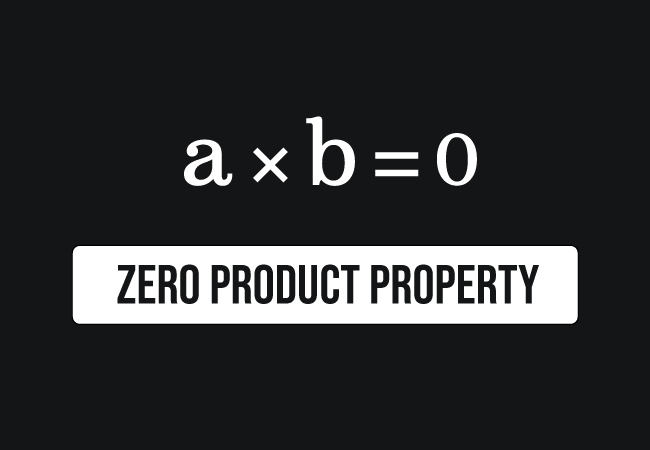# Zero Product property

## Property

If $a \times b \,=\, 0$ then $a \,=\, 0$ or $b \,=\, 0$

A product rule of two or more quantities when their product equals to zero, is called the zero product property.

## Introduction

Two or more quantities are involved in multiplication for expressing their product in mathematical form. The product of the quantities can be zero in a special case and it is possible only if one of the quantities is zero. Hence, the property is called the zero product property.Let $a$ and $b$ be two quantities in algebraic form. The multiplication of them is written mathematically as follows.

$a \times b$

Let’s assume that the product of the quantities $a$ and $b$ is zero.

$\implies$ $a \times b = 0$

In this case, there are two possibilities for their product to become zero.

$(1).\,\,\,$ If $a \,=\, 0$ then $0 \times b \,=\, 0$.

$(2).\,\,\,$ If $b \,=\, 0$ then $a \times 0 \,=\, 0$.

Therefore, the product of the quantities $a$ and $b$ is equal to zero when $a$ is equal to zero or $b$ is equal to zero. This rule is called the zero product property.

The zero product rule can also be applicable to more than two quantities.

$a \times b \times c \times \cdots$ $\,=\,$ $0$ where $a \,=\, 0$ or $b \,=\, 0$ or $c \,=\, 0$ and so on.

### Examples

The zero product property can be understood from the following numerical examples.

$(1).\,\,\,$ $0 \times 2 \,=\, 0$

$(2).\,\,\,$ $7 \times 0 \,=\, 7$

$(3).\,\,\,$ $5 \times 0 \times 6\,=\, 0$

Latest Math Topics
Jun 26, 2023
Jun 23, 2023

###### Math Questions

The math problems with solutions to learn how to solve a problem.

Learn solutions

Practice now

###### Math Videos

The math videos tutorials with visual graphics to learn every concept.

Watch now

###### Subscribe us

Get the latest math updates from the Math Doubts by subscribing us.